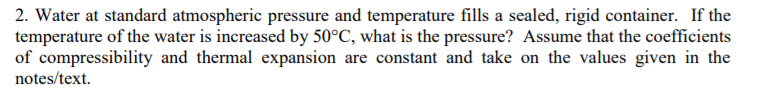# 2. Water at standard atmospheric pressure and temperature fills a sealed, rigid container. If thetemperature of the water is increased by 50°C, what is the pressure? Assume that the coefficientsof compressibility and thermal expansion are constant and take on the values given in thenotes/text

Questionhelp_outlineImage Transcriptionclose2. Water at standard atmospheric pressure and temperature fills a sealed, rigid container. If the temperature of the water is increased by 50°C, what is the pressure? Assume that the coefficients of compressibility and thermal expansion are constant and take on the values given in the notes/text fullscreen
check_circle

Step 1

Temperature of the water will be 50C

Assume the general parameters as P=pressure, V=volume,T=temperature

Step 2

Formula for coefficient of compressibility is

Step 3

Then the formula for coefficient of...

### Want to see the full answer?

See Solution

#### Want to see this answer and more?

Solutions are written by subject experts who are available 24/7. Questions are typically answered within 1 hour.*

See Solution
*Response times may vary by subject and question.
Tagged in

### Mechanical Engineering